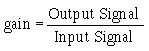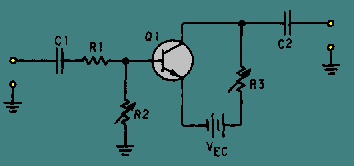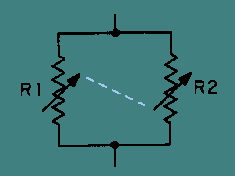amplifier can be constructed, you may be wondering about radio-frequency (rf) amplifiers. Do they use the same techniques? Are they just another type of broadband amplifier? The answer to both questions is "no." Radio-frequency amplifiers use different techniques than video amplifiers and are very different from them. ">Custom SearchNow that you have seen the way in which a broadband, or video, amplifier can be constructed, you may be wondering about radio-frequency (rf) amplifiers. Do they use the same techniques? Are they just another type of broadband amplifier?

The answer to both questions is "no." Radio-frequency amplifiers use different techniques than video amplifiers and are very different from them.

Before you study the specific techniques used in rf amplifiers, you should review some information on the relationship between the input and output impedance of an amplifier and the gain of the amplifier stage.

AMPLIFIER INPUT/OUTPUT IMPEDANCE AND GAIN

You should remember that the gain of a stage is calculated by using the input and output signals. The formula used to calculate the gain of a stage is:Voltage gain is calculated using input and output voltage; current gain uses input and output current; and power gain uses input and output power. For the purposes of our discussion, we will only be concerned with voltage gain.

Figure 2-11 shows a simple amplifier circuit with the input- and output-signal-developing impedances represented by variable resistors. In this circuit, C1 and C2 are the input and output coupling capacitors. R1 represents the impedance of the input circuit. R2 represents the input-signal-developing impedance, and R3 represents the output impedance.

Figure 2-11. - Variable input and output impedances.R1 and R2 form a voltage-divider network for the input signal. When R2 is increased in value, the input signal to the transistor (Q1) increases. This causes a larger output signal, and the gain of the stage increases.

Now look at the output resistor, R3. As R3 is increased in value, the output signal increases. This also increases the gain of the stage.

As you can see, increasing the input-signal-developing impedance, the output impedance, or both will increase the gain of the stage. Of course there are limits to this process. The transistor must not be overdriven with too high an input signal or distortion will result.

With this principle in mind, if you could design a circuit that had maximum impedance at a specific frequency (or band of frequencies), that circuit could be used in an rf amplifier. This FREQUENCY-DETERMINING NETWORK could be used as the input-signal-developing impedance, the output impedance, or both. The rf amplifier circuit would then be as shown in figure 2-12.

Figure 2-12. - Semiblock diagram of rf amplifier.In this "semi-block" diagram, C1 and C2 are the input and output coupling capacitors. R1 represents the impedance of the input circuit. The blocks marked FDN represent the frequency-determining networks. They are used as input-signal-developing and output impedances for Q1.

FREQUENCY-DETERMINING NETWORK FOR AN RF AMPLIFIER

What kind of circuit would act as a frequency-determining network? In general, a frequency-determining network is a circuit that provides the desired response at a particular frequency. This response could be maximum impedance or minimum impedance; it all depends on how the frequency-determining network is used. You will see more about frequency-determining networks in NEETS, module 9 - Introduction to Wave-Generation and Shaping Circuits. As you have seen, the frequency-determining network needed for an rf amplifier should have maximum impedance at the desired frequency.

Before you are shown the actual components that make up the frequency-determining network for an rf amplifier, look at figure 2-13, which is a simple parallel circuit. The resistors in this circuit are variable and are connected together (ganged) in such a way that as the resistance of R1 increases, the resistance of R2 decreases, and vice versa.

Figure 2-13. - Parallel variable resistors (ganged).If each resistor has a range from 0 to 200 ohms, the following relationship will exist between the individual resistances and the resistance of the network (RT). (All values are in ohms, RT rounded off to two decimal places. These are selected values; there are an infinite number of possible combinations.)

 R1 R2 RT 0 200 0.00 10 190 9.50 25 175 21.88 50 150 37.50 75 125 46.88 100 100 50.00 125 75 46.88 150 50 37.50 175 25 21.88 190 10 9.50 200 0 0.00

As you can see, this circuit has maximum resistance (RT) when the individual resistors are of equal value. If the variable resistors represented impedances and if components could be found that varied their impedance in the same way as the ganged resistors in figure 2-13 , you would have the frequency-determining network needed for an rf amplifier.

There are components that will vary their impedance (reactance) like the ganged resistors. As you know, the reactance of an inductor and a capacitor vary as frequency changes. As frequency increases, inductive reactance increases, and capacitive reactance decreases.

At some frequency, inductive and capacitive reactance will be equal. That frequency will depend upon the value of the inductor and capacitor. If the inductor and capacitor are connected as a parallel LC circuit, you will have the ideal frequency-determining network for an rf amplifier.

The parallel LC circuit used as a frequency-determining network is called a TUNED CIRCUIT. This circuit is "tuned" to give the proper response at the desired frequency by selecting the proper values of inductance and capacitance. A circuit using this principle is shown in figure 2-14 which shows an rf amplifier with parallel LC circuits used as frequency-determining networks. This rf amplifier will only be effective in amplifying the frequency determined by the parallel LC circuits.

Figure 2-14. - Simple rf amplifier.In many electronic devices, such as radio or television receivers or radar systems, a particular frequency must be selected from a band of frequencies. This could be done by using a separate rf amplifier for each frequency and then turning on the appropriate rf amplifier. It would be more efficient if a single rf amplifier could be "tuned" to the particular frequency as that frequency is needed. This is what happens when you select a channel on your television set or tune to a station on your radio. To accomplish this "tuning," you need only change the value of inductance or capacitance in the parallel LC circuits (tuned circuits).

In most cases, the capacitance is changed by the use of variable capacitors. The capacitors in the input and output portions of all the rf amplifier stages are ganged together in order that they can all be changed at one time with a single device, such as the tuning dial on a radio. (This technique will be shown on a schematic a little later in this chapter.)

Q.20 If the input-signal-developing impedance of an amplifier is increased, what is the effect on the gain?Q.21 If the output impedance of an amplifier circuit is decreased, what is the effect on the gain?Q.22 What is the purpose of a frequency-determining network in an rf amplifier?Q.23 Can a parallel LC circuit be used as the frequency-determining network for an rf amplifier?Q.24 How can the frequency be changed in the frequency-determining network?Integrated Publishing, Inc. - A (SDVOSB) Service Disabled Veteran Owned Small Business